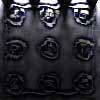#### You may also like### Fixing the Odds

You have two bags, four red balls and four white balls. You must put all the balls in the bags although you are allowed to have one bag empty. How should you distribute the balls between the two bags so as to make the probability of choosing a red ball as small as possible and what will the probability be in that case?### Scratch Cards

To win on a scratch card you have to uncover three numbers that add up to more than fifteen. What is the probability of winning a prize?### Escalator

At Holborn underground station there is a very long escalator. Two people are in a hurry and so climb the escalator as it is moving upwards, thus adding their speed to that of the moving steps. ... How many steps are there on the escalator?

# Stringing it Out

##### Age 14 to 16Challenge Level

$$T(x) = y$$ $$T(y) = xy$$This transformation has the following successive effect (starting with $x$): $$x \Rightarrow T(x) = y$$ $$y \Rightarrow T(y) = xy$$ $$xy \Rightarrow T(x)T(y) = yxy$$ $$yxy \Rightarrow T(y)T(x)T(y) = xyyxy$$ $$xyyxy \Rightarrow T(x)T(y)T(y)T(x)T(y) = \dots$$